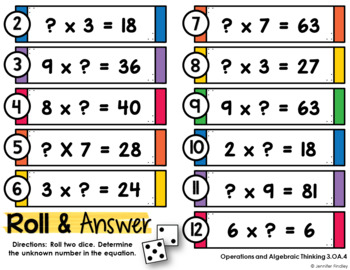Math Centers for 3rd Grade | 3rd Grade Algebraic Thinking Math CentersSubject
Resource Type
Product Rating
File Type

PDF (Acrobat) Document File

4 MB|40 pages
Share
Product Description

Want a fun, easy way to review the Common Core Math Skills? This is for you! This resource contains 13 ready to use, self-checking math center games for the Operations and Algebraic Thinking Common Core Standards for 3rd Grade.

I have included a color version of the game and another version of the game without as much color if you do not want to use your color ink. An answer key is included so the students can check their answers. I have also labeled the games with A, B, C, D, E so you can keep track of the different games and organize them.

This can be a 2, 3 or 4 player game! The students can even play this independently. The students roll two dice, add up their number, and then find their roll on the game board. They answer the question, check their answer on the included answer sheet,and initial their square if they get it correct. Super easy and fun! I have included two different directions pages and a blank directions page for you to choose how you want your students to play the game.

The games are:

1. Drawing arrays to solve multiplication problems.

2. Writing story problems to match multiplication problems.

3. Using models to solve division problems.

4. Writing story problems to match division problems.

5. Solve one step multiplication and division word problems.

6. Determining the unknown number in a multiplication problem.

7. Determining the unknown number in a division problem.

8. Properties of multiplication

9. Using related multiplication problems to solve division problems.

10. Solving groups of multiplication facts (to build fluency)

11. Solving groups of division facts (to build fluency)

12. Solve two step word problems.

13. Completing addition and multiplication patterns with tables.

Covers these standards:

3.OA.1 Interpret products of whole numbers, e.g., interpret 5 × 7 as the total number of objects in 5 groups of 7 objects each.

3.OA.2 Interpret whole-number quotients of whole numbers, e.g., interpret 56 ÷ 8 as the number of objects in each share when 56 objects are partitioned equally into 8 shares, or as a number of shares when 56 objects are partitioned into equal shares of 8 objects each.

3.OA.3 Use multiplication and division within 100 to solve word problems in situations involving equal groups, arrays, and measurement quantities, e.g., by using drawings and equations with a symbol for the unknown number to represent the problem.

3.OA.4 Determine the unknown whole number in a multiplication or division equation relating three whole numbers.

3.OA.5 Apply properties of operations as strategies to multiply and divide.

3.OA.6 Understand division as an unknown-factor problem.

3.OA.7 Fluently multiply and divide within 100, using strategies such as the relationship between multiplication and division.

3.OA.8 Solve two-step word problems using the four operations.

3.OA.9 Identify arithmetic patterns (including patterns in the addition table or multiplication table), and explain them using properties of operations.

You may also like:

Total Pages
40 pages